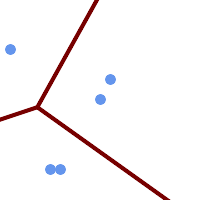## Name

ST_VoronoiLines — Returns the boundaries of the Voronoi diagram of the vertices of a geometry.

## Synopsis

`geometry ST_VoronoiLines(` geometry geom , float8 tolerance = 0.0 , geometry extend_to = NULL `)`;

## 설명

Computes a two-dimensional Voronoi diagram from the vertices of the supplied geometry and returns the boundaries between cells in the diagram as a MultiLineString. Returns null if input geometry is null. Returns an empty geometry collection if the input geometry contains only one vertex. Returns an empty geometry collection if the `extend_to` envelope has zero area.

선택할 수 있는 파라미터:

• `tolerance`: The distance within which vertices will be considered equivalent. Robustness of the algorithm can be improved by supplying a nonzero tolerance distance. (default = 0.0)

• `extend_to`: If present, the diagram is extended to cover the envelope of the supplied geometry, unless smaller than the default envelope (default = NULL, default envelope is the bounding box of the input expanded by about 50%).

GEOS 모듈로 실행

2.3.0 버전부터 사용할 수 있습니다.

## 예시Voronoi diagram lines, with tolerance of 30 units

```SELECT ST_VoronoiLines(
'MULTIPOINT (50 30, 60 30, 100 100,10 150, 110 120)'::geometry,
30) AS geom;
```
```ST_AsText output
MULTILINESTRING((135.555555555556 270,36.8181818181818 92.2727272727273),(36.8181818181818 92.2727272727273,-110 43.3333333333333),(230 -45.7142857142858,36.8181818181818 92.2727272727273))
```